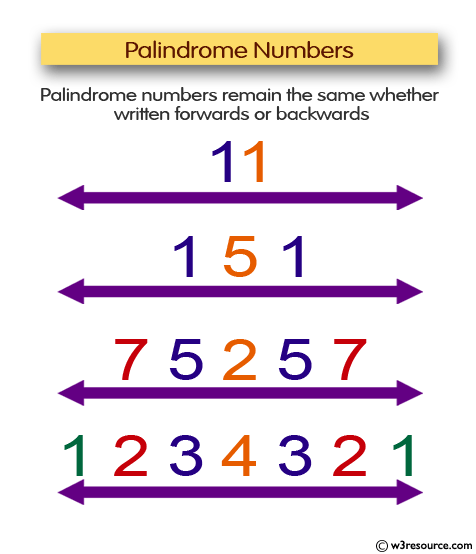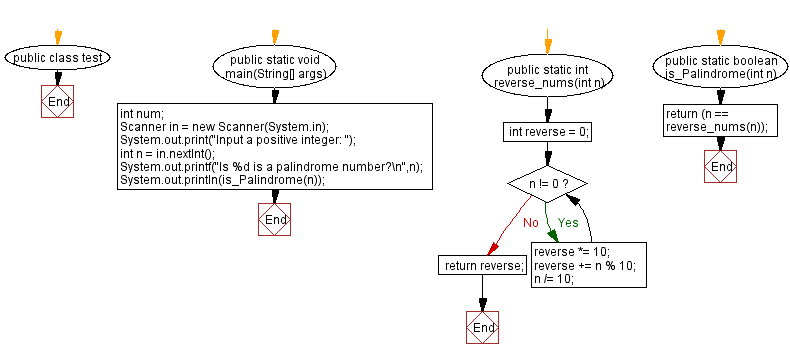﻿ Java: Check if a positive number is a palindrome or not# Java Exercises: Check if a positive number is a palindrome or not

## Java Basic: Exercise-115 with Solution

Write a Java program to check if a positive number is a palindrome or not.

Pictorial Presentation:Sample Solution:

Java Code:

``````import java.util.*;
public class test {
public static void main(String[] args)
{
int num;
Scanner in = new Scanner(System.in);
System.out.print("Input a positive integer: ");
int n = in.nextInt();
System.out.printf("Is %d is a palindrome number?\n",n);
System.out.println(is_Palindrome(n));
}
public static int reverse_nums(int n) {
int reverse = 0;
while (n != 0) {
reverse *= 10;
reverse += n % 10;
n /= 10;
}
return reverse;
}
public static boolean is_Palindrome(int n) {
return (n == reverse_nums(n));
}
}
```
```

Sample Output:

```Input a positive integer: 151
Is 151 is a palindrome number?
true
```

Flowchart:Java Code Editor:

What is the difficulty level of this exercise?

Test your Programming skills with w3resource's quiz.

﻿

## Java: Tips of the Day

countOccurrences

Counts the occurrences of a value in an array.

Use Arrays.stream().filter().count() to count total number of values that equals the specified value.

```public static long countOccurrences(int[] numbers, int value) {
return Arrays.stream(numbers)
.filter(number -> number == value)
.count();
}
```

Ref: https://bit.ly/3kCAgLb